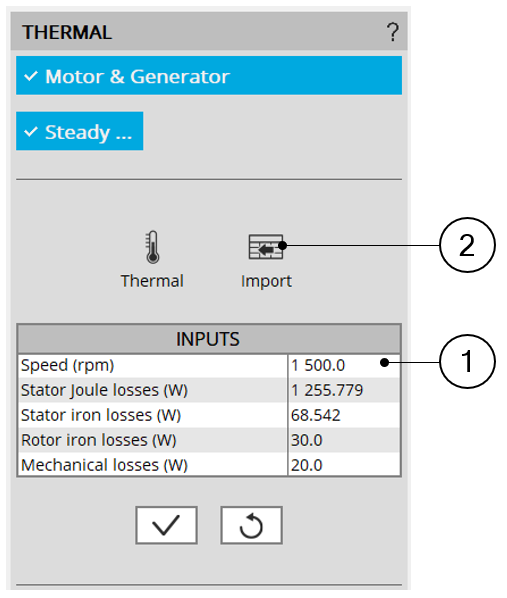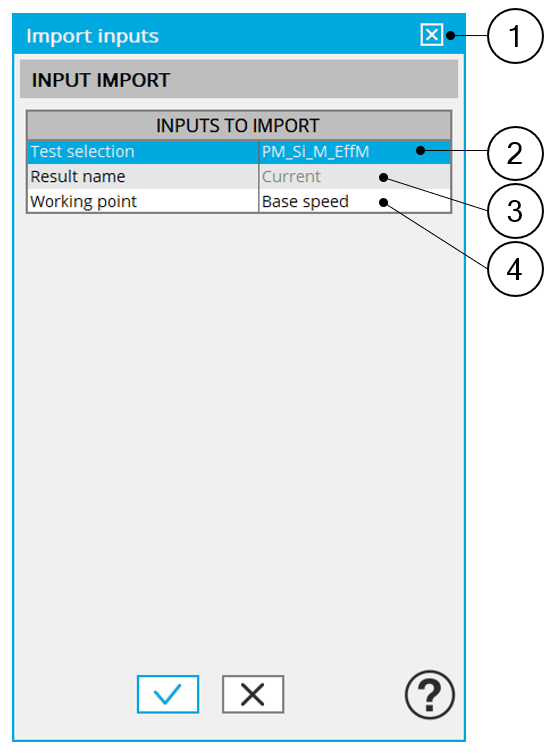# Inputs

## 1. Introduction

The main inputs of these test correspond to a set of losses to be considered for evaluating the thermal behavior of the machine.

## 2.1 Speed

The speed of the machine to be considered.

## 2.2 Set of losses

The losses to be defined are the following ones:

• Stator Joule losses
• Stator iron losses
• Rotor iron losses
• Mechanical losses

## 2.3 Input import

The set of inputs can be imported from another test already performed in Motor Factory Test environment.

It can be current results or saved test results.How to define a set of losses 1 Fill in the table to define the speed and all the losses to be considered 2 Click on the button “import” to import inputs corresponding to a solved test. All existing data of the selected test will be imported into the inputs of the thermal characterization. The data which does not exist in the outputs of the selected test are set to 0.Dialog box to select the test from which a set of losses must be imported 1 Opened dialog box after having clicked on the button “import” on the main panel. 2 Selection of the test. Only solved tests are available in the drop-down list. 3 Current tests as well as saved test can be selected. 4 When several working points are computed in the selected test, each of them can be selected to consider the corresponding losses.
Note: The imported data are the output data directly shown in the considered solved test.

For some tests, some values are not defined (like for instance the rotor iron losses). In that case, the corresponding values are set to 0 in the thermal characterization input table.